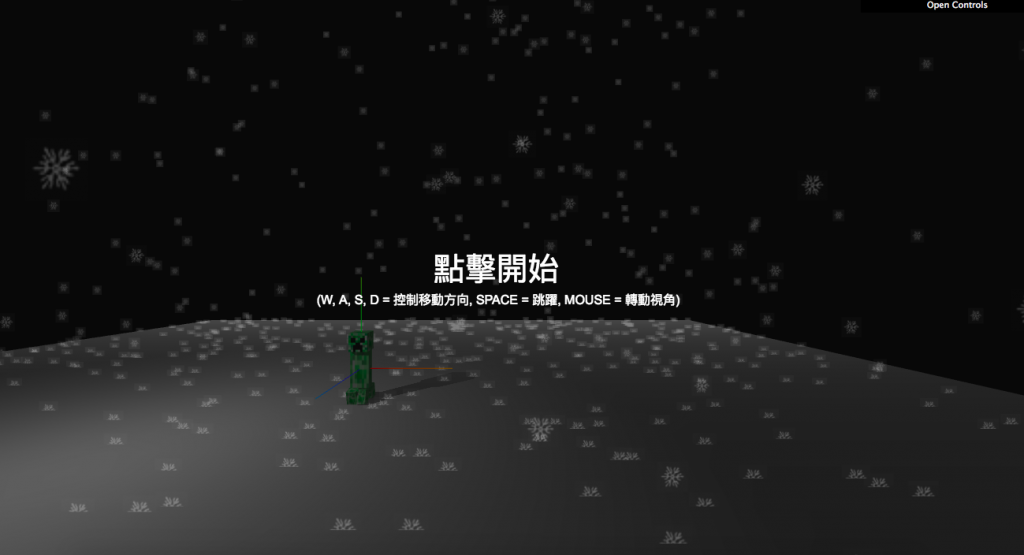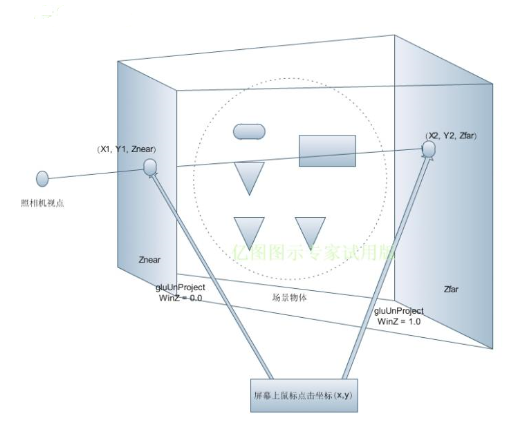#2019 iT 邦幫忙鐵人賽DAY 23
3
Modern Web

## 用 Three.js 來當個創世神 (22)：專案實作#11 - 使用 PointerLockControls 實現射擊遊戲視角 Part.2Photo by Michael Sum on Unsplash

## 今日目標## 程式解析

### 1. 初始化 Raycaster 做為跳躍的碰撞偵測

``````raycaster = new THREE.Raycaster(
new THREE.Vector3(), // 射線起點向量
new THREE.Vector3(0, -1, 0), // 射線投射方向向量
0, // 投射近點
10 // 投射遠點
)
``````Photo by mooonx on segmentfault

### 2. 更新鼠標控制器畫面

#### (1) 基本設定

``````let prevTime = Date.now() // 初始時間
let velocity = new THREE.Vector3() // 移動速度向量
let direction = new THREE.Vector3() // 移動方向向量

function pointerLockControlsRender() {
if (controls.isLocked === true) {
// 計算時間差
const time = Date.now()
const delta = (time - prevTime) / 1000 // 大約為 0.016
...
prevTime = time
}
}
``````

#### (2) 跳躍

``````// Raycaster 複製控制器的位置
raycaster.ray.origin.copy(controls.getObject().position)

// 使用 Raycaster 判斷腳下是否與場景中物體相交
const intersections = raycaster.intersectObjects(scene.children, true)
const onObject = intersections.length > 0

// 判斷是否停在地面上
if (onObject === true) {
velocity.y = Math.max(0, velocity.y)
canJump = true
}

controls.getObject().translateY(velocity.y * delta)

// 控制器下墜超過 -2000 則重置位置
if (controls.getObject().position.y < -2000) {
velocity.y = 0
controls.getObject().position.set(10, 100, 60)
canJump = true
}
``````

``````case 32: // space
if (canJump === true) velocity.y += 350 // 跳躍高度
canJump = false
break
``````

#### (3) 移動

``````// 設定每次更新時速度
velocity.x -= velocity.x * 10.0 * delta
velocity.z -= velocity.z * 10.0 * delta

// 判斷按鍵朝什麼方向移動，並設定對應方向速度變化
direction.z = Number(moveForward) - Number(moveBackward)
direction.x = Number(moveLeft) - Number(moveRight)

// 向量正規化（長度為 1），確保每個方向保持一定移動量
direction.normalize()

// 根據移動方向變更速度值
if (moveForward || moveBackward) velocity.z -= direction.z * 400.0 * delta
if (moveLeft || moveRight) velocity.x -= direction.x * 400.0 * delta

// 根據速度值移動控制器位置
controls.getObject().translateX(velocity.x * delta)
controls.getObject().translateZ(velocity.z * delta)
``````

## 參考資料

### 1 則留言

0

``````if (canJump === true) velocity.y += 350 // 跳躍高度
``````